# K-Means Clustering in Python

01 October 2017 — Written by Mubaris NK

Clustering is a type of Unsupervised learning. This is very often used when you don't have labeled data. K-Means Clustering is one of the popular clustering algorithm. The goal of this algorithm is to find groups(clusters) in the given data. In this post we will implement K-Means algorithm using Python from scratch.

## K-Means Clustering

K-Means is a very simple algorithm which clusters the data into K number of clusters. The following image from PyPR is an example of K-Means Clustering.### Use Cases

K-Means is widely used for many applications.

• Image Segmentation
• Clustering Gene Segementation Data
• News Article Clustering
• Clustering Languages
• Species Clustering
• Anomaly Detection

### Algorithm

Our algorithm works as follows, assuming we have inputs $x_1, x_2, x_3, ..., x_n$ and value of K

• Step 1 - Pick K random points as cluster centers called centroids.
• Step 2 - Assign each $x_i$ to nearest cluster by calculating its distance to each centroid.
• Step 3 - Find new cluster center by taking the average of the assigned points.
• Step 4 - Repeat Step 2 and 3 until none of the cluster assignments change.The above animation is an example of running K-Means Clustering on a two dimensional data.

#### Step 1

We randomly pick K cluster centers(centroids). Let's assume these are $c_1, c_2, ..., c_k$, and we can say that;

$C = {c_1, c_2,..., c_k}$

$C$ is the set of all centroids.

#### Step 2

In this step we assign each input value to closest center. This is done by calculating Euclidean(L2) distance between the point and the each centroid.

$\arg \min_{c_i \in C} dist(c_i, x)^2$

Where $dist(.)$ is the Euclidean distance.

#### Step 3

In this step, we find the new centroid by taking the average of all the points assigned to that cluster.

$c_i = \frac{1}{\lvert S_i \rvert}\sum_{x_i \in S_i} x_i$

$S_i$ is the set of all points assigned to the $i$th cluster.

#### Step 4

In this step, we repeat step 2 and 3 until none of the cluster assignments change. That means until our clusters remain stable, we repeat the algorithm.

### Choosing the Value of K

We often know the value of K. In that case we use the value of K. Else we use the Elbow Method.We run the algorithm for different values of K(say K = 10 to 1) and plot the K values against SSE(Sum of Squared Errors). And select the value of K for the elbow point as shown in the figure.

### Implementation using Python

The dataset we are gonna use has 3000 entries with 3 clusters. So we already know the value of K.

Checkout this Github Repo for full code and dataset.

We will start by importing the dataset.

%matplotlib inline
from copy import deepcopy
import numpy as np
import pandas as pd
from matplotlib import pyplot as plt
plt.rcParams['figure.figsize'] = (16, 9)
plt.style.use('ggplot')
# Importing the dataset
print(data.shape)
data.head()
(3000, 2)
V1 V2
0 2.072345 -3.241693
1 17.936710 15.784810
2 1.083576 7.319176
3 11.120670 14.406780
4 23.711550 2.557729
# Getting the values and plotting it
f1 = data['V1'].values
f2 = data['V2'].values
X = np.array(list(zip(f1, f2)))
plt.scatter(f1, f2, c='black', s=7)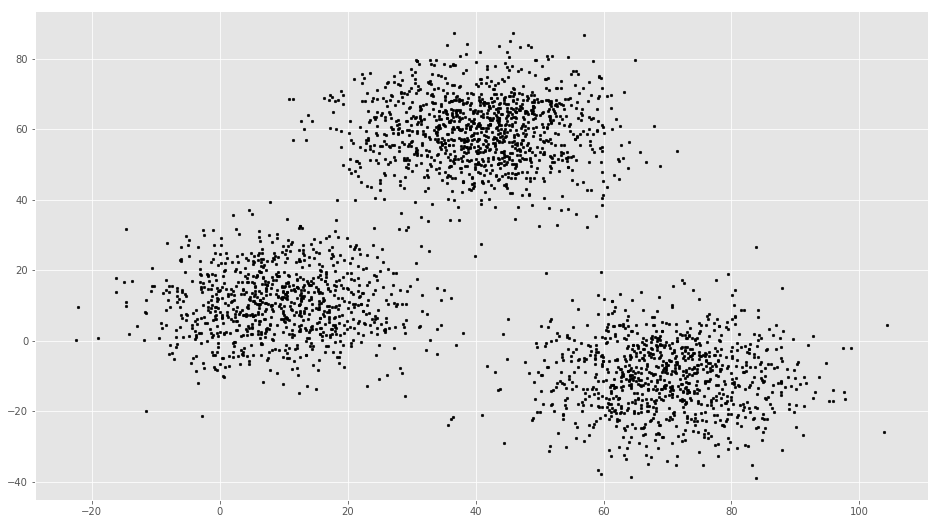# Euclidean Distance Caculator
def dist(a, b, ax=1):
return np.linalg.norm(a - b, axis=ax)
# Number of clusters
k = 3
# X coordinates of random centroids
C_x = np.random.randint(0, np.max(X)-20, size=k)
# Y coordinates of random centroids
C_y = np.random.randint(0, np.max(X)-20, size=k)
C = np.array(list(zip(C_x, C_y)), dtype=np.float32)
print(C)
[[ 11.  26.]
[ 79.  56.]
[ 79.  21.]]
# Plotting along with the Centroids
plt.scatter(f1, f2, c='#050505', s=7)
plt.scatter(C_x, C_y, marker='*', s=200, c='g')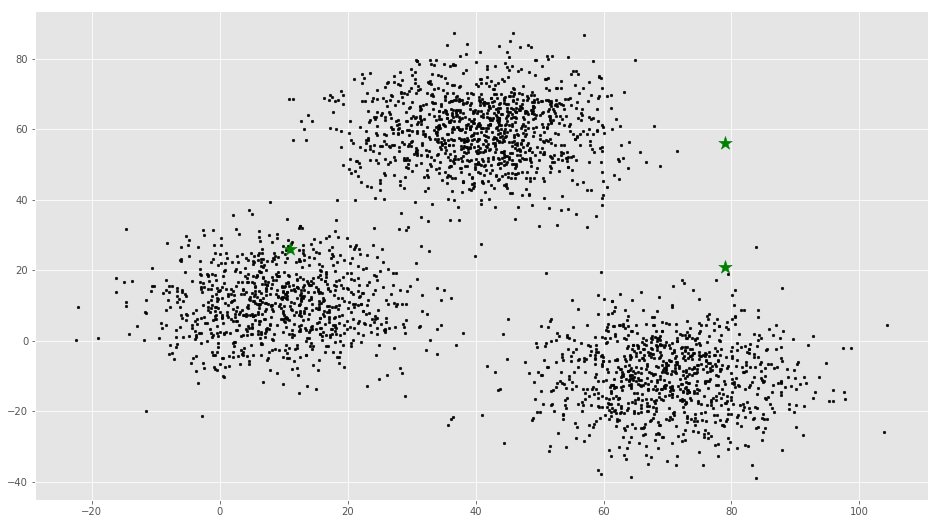# To store the value of centroids when it updates
C_old = np.zeros(C.shape)
# Cluster Lables(0, 1, 2)
clusters = np.zeros(len(X))
# Error func. - Distance between new centroids and old centroids
error = dist(C, C_old, None)
# Loop will run till the error becomes zero
while error != 0:
# Assigning each value to its closest cluster
for i in range(len(X)):
distances = dist(X[i], C)
cluster = np.argmin(distances)
clusters[i] = cluster
# Storing the old centroid values
C_old = deepcopy(C)
# Finding the new centroids by taking the average value
for i in range(k):
points = [X[j] for j in range(len(X)) if clusters[j] == i]
C[i] = np.mean(points, axis=0)
error = dist(C, C_old, None)
colors = ['r', 'g', 'b', 'y', 'c', 'm']
fig, ax = plt.subplots()
for i in range(k):
points = np.array([X[j] for j in range(len(X)) if clusters[j] == i])
ax.scatter(points[:, 0], points[:, 1], s=7, c=colors[i])
ax.scatter(C[:, 0], C[:, 1], marker='*', s=200, c='#050505')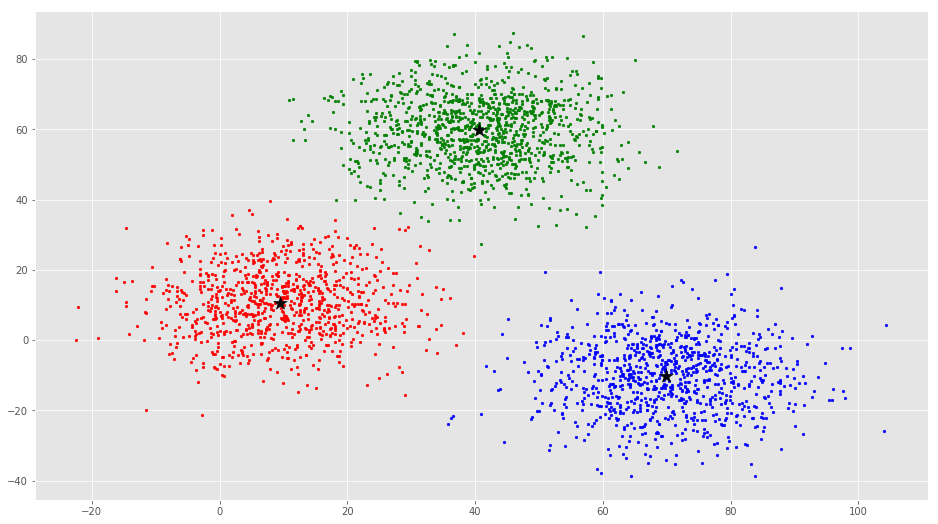From this visualization it is clear that there are 3 clusters with black stars as their centroid.

If you run K-Means with wrong values of K, you will get completely misleading clusters. For example, if you run K-Means on this with values 2, 4, 5 and 6, you will get the following clusters.Now we will see how to implement K-Means Clustering using scikit-learn

### The scikit-learn approach

#### Example 1

We will use the same dataset in this example.

from sklearn.cluster import KMeans

# Number of clusters
kmeans = KMeans(n_clusters=3)
# Fitting the input data
kmeans = kmeans.fit(X)
# Getting the cluster labels
labels = kmeans.predict(X)
# Centroid values
centroids = kmeans.cluster_centers_
# Comparing with scikit-learn centroids
print(C) # From Scratch
print(centroids) # From sci-kit learn
[[  9.47804546  10.68605232]
[ 40.68362808  59.71589279]
[ 69.92418671 -10.1196413 ]]
[[  9.4780459   10.686052  ]
[ 69.92418447 -10.11964119]
[ 40.68362784  59.71589274]]

You can see that the centroid values are equal, but in different order.

#### Example 2

We will generate a new dataset using make_blobs function.

import numpy as np
import matplotlib.pyplot as plt
from mpl_toolkits.mplot3d import Axes3D
from sklearn.cluster import KMeans
from sklearn.datasets import make_blobs

plt.rcParams['figure.figsize'] = (16, 9)

# Creating a sample dataset with 4 clusters
X, y = make_blobs(n_samples=800, n_features=3, centers=4)
fig = plt.figure()
ax = Axes3D(fig)
ax.scatter(X[:, 0], X[:, 1], X[:, 2])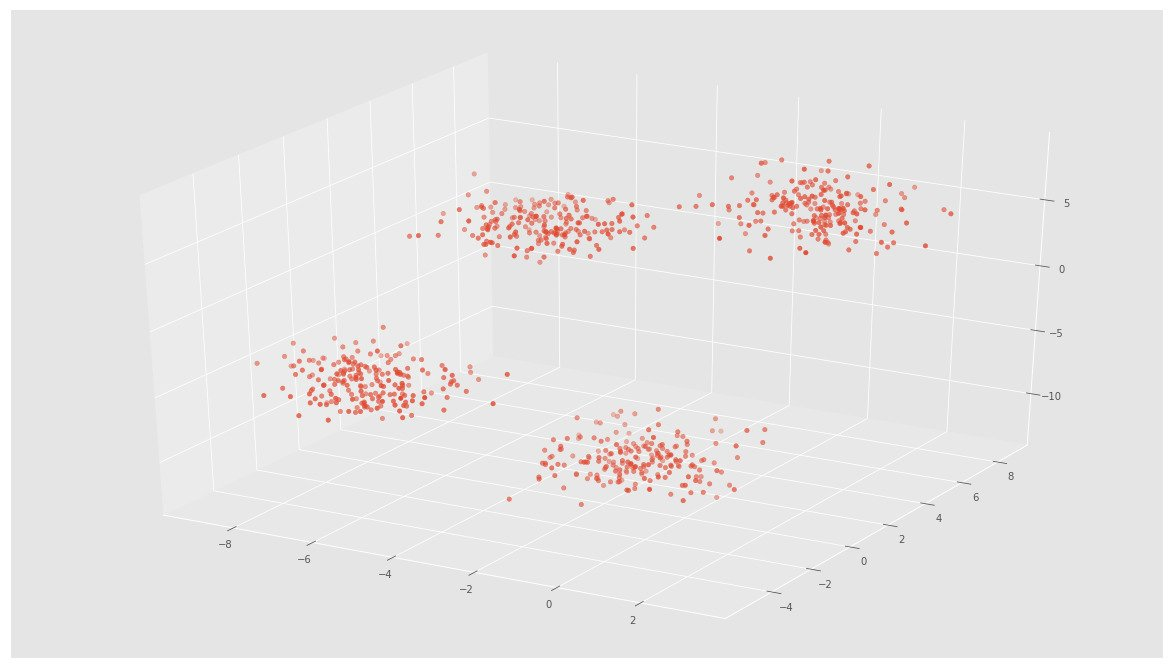# Initializing KMeans
kmeans = KMeans(n_clusters=4)
# Fitting with inputs
kmeans = kmeans.fit(X)
# Predicting the clusters
labels = kmeans.predict(X)
# Getting the cluster centers
C = kmeans.cluster_centers_
fig = plt.figure()
ax = Axes3D(fig)
ax.scatter(X[:, 0], X[:, 1], X[:, 2], c=y)
ax.scatter(C[:, 0], C[:, 1], C[:, 2], marker='*', c='#050505', s=1000)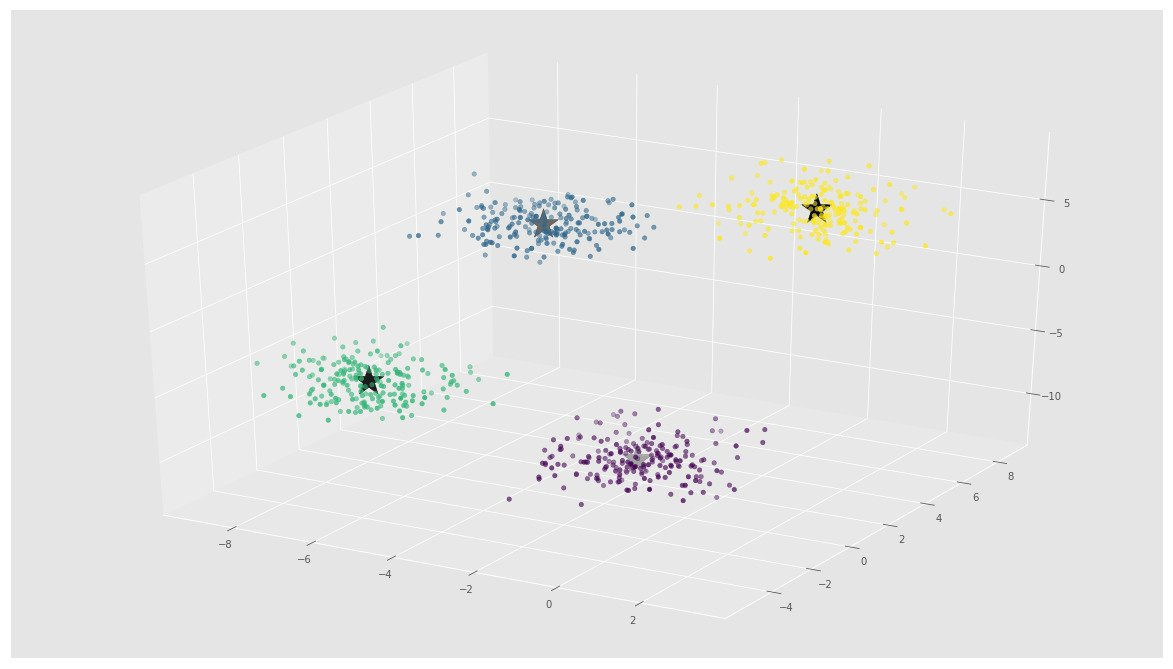In the above image, you can see 4 clusters and their centroids as stars. scikit-learn approach is very simple and concise.

## More Resources

Checkout this Github Repo for full code and dataset.

## Conclusion

Even though it works very well, K-Means clustering has its own issues. That include:

• If you run K-means on uniform data, you will get clusters.
• Sensitive to scale due to its reliance on Euclidean distance.
• Even on perfect data sets, it can get stuck in a local minimum

Have a look at this StackOverflow Answer for detailed explanation.

Let me know if you found any errors and checkout this post on Hacker News